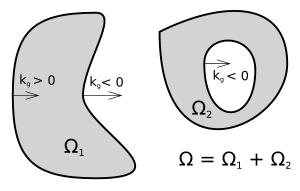# Gauss-Bonnet theorem facts for kids

Kids Encyclopedia FactsAn example of complex region where Gauss-Bonnet theorem can apply. Shows the sign of geodesic curvature.

The Gauss-Bonnet theorem is a theorem that connects the geometry of a shape with its topology. It is named after the two mathematicians Carl Friedrich Gauß (1777-1855) and Pierre Ossian Bonnet (1812-1872) who both found it, independently of one another. The Curvature of the shape is used, as well as its Euler characteristic.Gauss-Bonnet theorem Facts for Kids. Kiddle Encyclopedia.